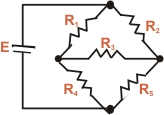×#### Thank you for registering.

One of our academic counsellors will contact you within 1 working day.

Click to Chat

1800-1023-196

+91-120-4616500

CART 0

• 0

MY CART (5)

Use Coupon: CART20 and get 20% off on all online Study Material

ITEM
DETAILS
MRP
DISCOUNT
FINAL PRICE
Total Price: Rs.

There are no items in this cart.
Continue Shopping• Complete Physics Course - Class 11
• OFFERED PRICE: Rs. 2,968
• View Details

```Complicated Circuits

As the name suggests, complicated circuits are the circuits which are complex in the sense that they cannot be simplified by series and parallel combinations. As a result, Ohm’s Law cannot be used for solving such circuits. This kind of situation arises in two circumstances:

1. This can happen in the case when the circuit has more than one source of emf.

2. Complicated circuits can also arise when the resistors are attached in a complicated manner.

The circuits described in the above two categories are termed as complex or complicated circuits.

We further explain these two forms of circuits with the help of figures:

The figure given below demonstrates a circuit having two sources of emf E1 and E2 and three resistors. It is quite obvious that this circuit cannot be solved by series-parallel combinations because the resistors R1 and R3 are not in series as the same current does not flow between them.Now, consider another case where again it is not possible to solve the circuit using series and parallel combinations. Though, the circuit contains only one source of emf, yet because the resistors R1 and R2 are neither in series nor parallel so they cannot be solved by series or parallel combinations. The same reason applies to all other pairs of resistors.In order to solve such kinds of circuits, we have the Kirchhoff’s laws. These laws describe the relationship between currents and voltages of diverse branches of electric circuit. These laws were derived by Guatov Robert Kirchhoff and named after him. They are basically useful in determining the resistance or impedance of a network.

The Kirchhoff’s laws can also be formulated in two ways:

1. Kirchhoff’s Current Law (KCL): This is the law of conservation of charge and states that the algebraic sum of currents at any junction of a circuit is zero. i.e. at any junction, the current flowing in should be equal to the current flowing out.

ΣIi = 0.

The law implies that the net result of all currents in a circuit is zero. If all the currents entering in the junction are considered to be positive then by convention all the currents leaving the circuit are negative so that the on addition we get a result of zero.

View the following video for more on the Kirchhoff’s Current Law

2. Kirchhoff’s Voltage Law (KVL):

This law is also abbreviated as KVL on complicated circuit. Kirchhoff’s voltage law states that in closed mesh (any closed loop contained in a circuit), the algebraic sum of all the voltage drops encountered is zero if there is no source of emf enclosed in the mesh or equal to the magnitude of enclosed emf.

Σ IiRi= Σ ∈i

KVL is a fundamental law and as important as the law of Conservation of energy in mechanics, and in fact, KVL is also conservation of electrical energy. The analysis of any circuit is initiated with KVL and KCL. KCL and KVL hold good always and are of immense importance.Application of Kirchhoff's Laws to Circuits

The Kirchhoff’s Current Law can be applied at various junctions in the circuit to find the distribution of current. After applying this law, the Kirchhoff voltage law can be applied to the different loops in the circuit so as to generate an algebraic equation for each loop.  Hence, it becomes quite simple to calculate the unknown currents, voltages and resistances by solving the formed equations.

DC Circuits: DC is an abbreviation for direct current. A DC electrical circuit consists of a source of DC electricity in addition to a conduction wire which connects the source terminal to some electrical device and then again back to another terminal to complete the circuit. DC electricity can exist only in the presence of DC circuit. Dc circuits may exist in series, parallel or in combination.Remark: The points to be remembered while analyzing a DC circuits are:

(a) In a circuit current always flows from a region of higher potential to a region of lower potential.

(b) Initially, the direction of flow of current may be assumed at random, provided the current distribution is made correctly. It does not matter whether voltage drop is taken to be negative or positive but it is compulsory to use the same convention for the whole analysis. After calculations are done, the value of current may turn out to negative and this just indicates that current flows in the direction opposite to that assumed initially.

(c) A voltage source always provides the same voltage to the circuit irrespective of the current flow. A current source always provides a steady current irrespective of the voltage drops in a circuit.

askIITians offers wide-ranging study material which covers all the topics of Physics of IIT JEE. The notes also contain questions on KVL and KCL complicated circuit with answers. DC circuit sums of JEE have also been included. It is important to master this topic in order to remain competitive in the JEE.

Related Resources:

Look into the Past Year Papers with Solutions to get a hint of the kinds of questions asked in the exam.

You can get the knowledge of Useful Books of Physics here.

To read more, Buy study materials of Current Electricity comprising study notes, revision notes, video lectures, previous year solved questions etc. Also browse for more study materials on Physics here.
```### Course Features

• 101 Video Lectures
• Revision Notes
• Previous Year Papers
• Mind Map
• Study Planner
• NCERT Solutions
• Discussion Forum
• Test paper with Video Solution